#### Vlookup函数最经典的10种用法

Excel函数有很多，很多函数用法小编都有给大家分享过，不过今天要给大家重磅介绍的是Vlookup函数最经典的10种用法，掌握它们，说不定工作上有意外惊喜哦!下面就一起来看下吧!

1、计算个人所得税

=(G28-3500)*VLOOKUP(G28-3500,C28:E34,2)-VLOOKUP(G28-3500,C28:E34,3)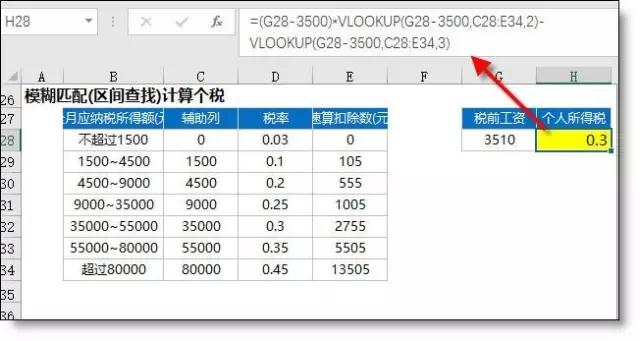2、查找个人信息

=VLOOKUP(\$B18,\$C\$7:\$H\$15,COLUMN(B1),0)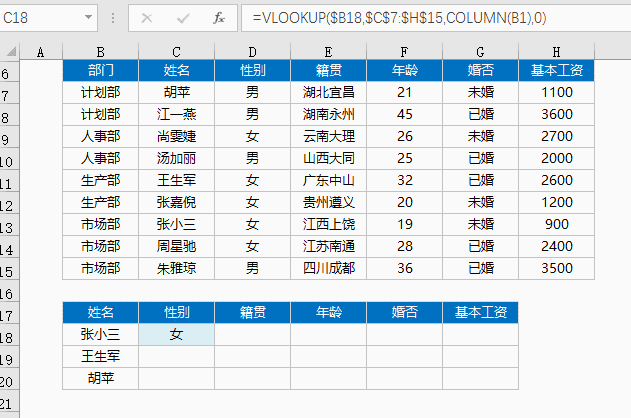3、合并两个表格

F2单元格公式

=VLOOKUP(B2,\$B\$14:\$F\$22,MATCH(F\$1,\$B\$13:\$F\$13,0))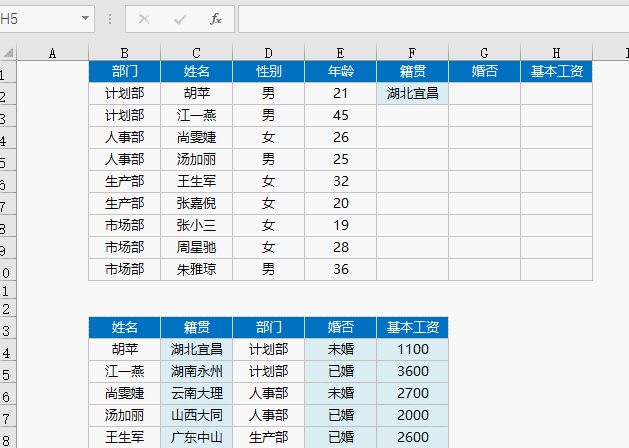4、让两个表格同类商品排序完全一样

F2单元格公式

=IFERROR(VLOOKUP(\$B2,\$B\$12:\$D\$16,COLUMN(A1),),"")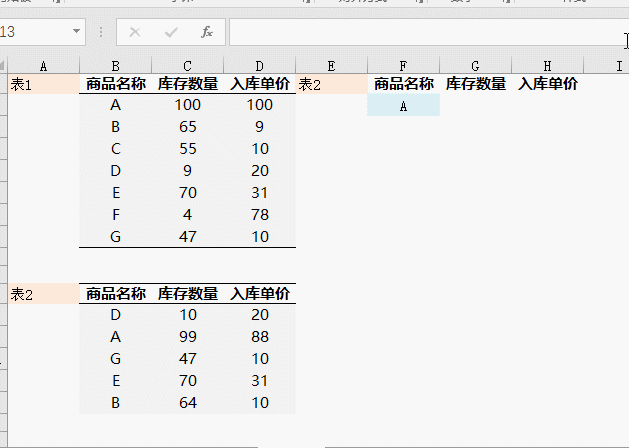5、模糊查找产品名称(包含查找)

=VLOOKUP("*"&F41&"*",\$B\$41:\$D\$47,3,0)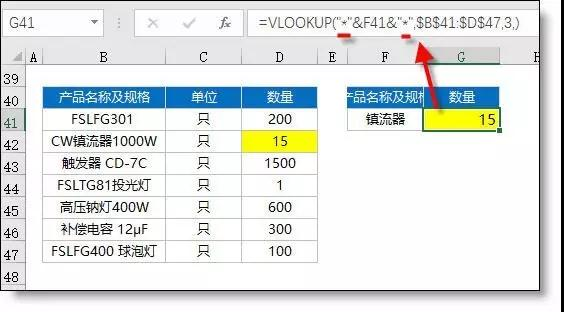6、带特殊符合的字符查找

=VLOOKUP(SUBSTITUTE(F52,"~","~~"),B\$52:C\$56,2,0)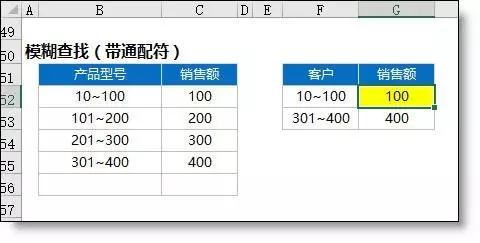7、逆向查找

=VLOOKUP(G61,IF({1,0},C61:C69,B61:B69),2,)

=INDEX(B60:B69,MATCH(G61,C60:C69,0))8、Vlookup多条件查找

A112 =B112&C112

H112 =VLOOKUP(F112&G112,A112:D120,4,0)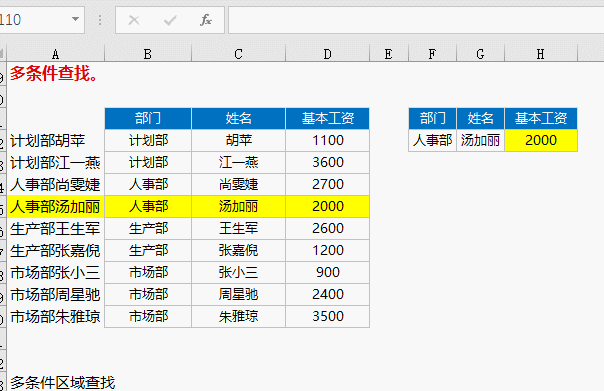9、16年与17年单价核对，表格核对(带通配符)，结果显示多N或少N

=TEXT(VLOOKUP(SUBSTITUTE(B73,"*","~*"),\$F\$73:\$G\$92,2,)-C73,"少0.00;多0.00;")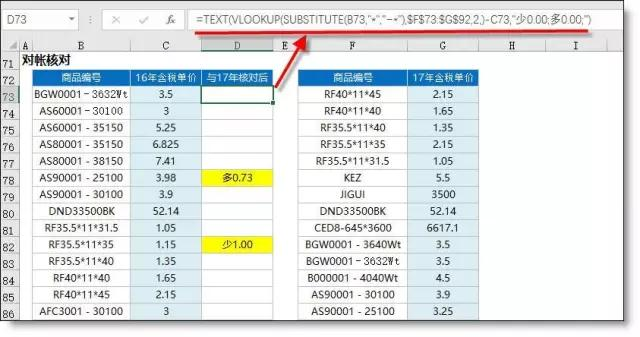10、库存单价的监控。销售单价>库存单价时填充红色背景=H2>VLOOKUP(F2,A:C,3,0)